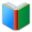Words for students of 6th Grade, the page is for math words (List 1), which are essential vocabulary for 6th Grade academic classes.
 Free Online Vocabulary TestK12, SAT, GRE, IELTS, TOEFL
 Actions upon current list

 All lists of current grdae
Spelling Words:

Arts Words:

Social Words:

Math Words:

Science Words:

Literature Words:The SwineherdThe Red ShoesA Study In Scarlet
 6th Grade Cards: Math (click the card to toggle it.)

(You can also click each card to show or hide its content.)
the number that is going to be raised to a power
boundary line of a circle, figure, area, or object
complete; consummate; make perfect
change something into another form; transform
coordinate system that specifies each point uniquely in a plane by a pair of numerical coordinates
length of straight line passing through the center of a circle and connecting two points on the circumference
judge; examine and judge carefully; appraise
the resolution of an entity into factors such that when multiplied together they give the original entity
a decimal numeral that does not terminate in an infinite sequence
combines numbers, variables, or constants using operators
the ratio of a circle's circumference to its diameter; equal to 3.14159265358979323846...
a two-dimensional shape
the prime factors of a quantity are all of the prime quantities that will exactly divide the given quantity
right line drawn or extending from the center of a circle to the periphery
concerning each of two or more persons or things; exchangeable; interacting
the action of following in order; serial arrangement in which things follow in logical order or a recurrent pattern
the quality of being similar
make simple; make less complex; make clear by giving the explanation for
unit used to measure area
the act of putting one thing or person in the place of another; an event in which one thing is substituted for another